# Texas Go Math Grade 3 Lesson 8.6 Answer Key Two-Step Multiplication Problems

Refer to our Texas Go Math Grade 3 Answer Key Pdf to score good marks in the exams. Test yourself by practicing the problems from Texas Go Math Grade 3 Lesson 8.6 Answer Key Two-Step Multiplication Problems.

## Texas Go Math Grade 3 Lesson 8.6 Answer Key Two-Step Multiplication Problems

Essential Question
How can you use the strategy make a table to solve one-step and two-step multiplication problems?

Unlock the Problem
Scott has a stamp album. Some pages have I stamp on them, and other pages have 2 stamps on them. If Scott has 18 stamps, show how many different ways he could put them in the album. Use the graphic organizer below to solve the problem.

What do I need to find?

What information am I given?

Scott has ___ stamps. Some album pages have __ stamp on them, and the other pages have ___ stamps.

Plan
What is my plan or strategy?
I will make a ___ showing all the different ways of arranging the stamps in the album.

Solve
Make a table to show the number of pages with 1 stamp and with 2 stamps. Each row must equal ___, the total number of stamps.
Pages with Pages with Total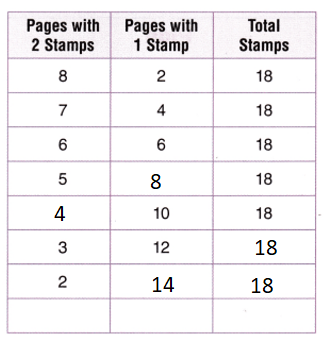Explanation:
Pages with 2 stamps + Pages with 1 stamp = Total stamps.
2(5) + ?? = 18
=> 10 + ?? = 18.
=> ?? = 18 – 10
=> ?? = 8.
??(2) + 10 = 18.
=> ??(2) = 18 – 10
=> ?? = 8 ÷ 2
=> ?? = 4.
3 (2) + 12 = 6 + 12 = 18.
2(2) + ?? = 18.
=> 4 + ?? = 18
=> ?? = 18 – 4
=> ?? = 14.

Try Another Problem
What if Scott bought 3 more stamps and now has 21 stamps? Some album pages have 1 stamp and some pages have 2 stamps. Show how many different ways he could put the odd number of stamps in the album.

What do I need to find?

What information am I given?

Plan
What is my plan or strategy?

Solve

So, there are ___ different ways.
So, there are 2 different ways.

Explanation:One way:
Pages with 2 stamps + Pages with 1 stamp = Total stamps.
Add 2 stamps in pages with 2 stamps and 1 in pages with 2 stamp = Total stamps.Other way:
Pages with 2 stamps + Pages with 1 stamp = Total stamps.
Pages with 2 stamps + Add 3 pages to Pages with 1 stamp = Total stamps.Question 2.
What patterns do you see in this table?
Patterns saw in this table is if pages with 2 pages with 1 stamps increased by 2 then the pages with 1 stamp also increased by 2.

Explanation:
It is noticed that if pages with 2 pages with 1 stamps increased by 2 then the pages with 1 stamp also increased by 2.

Math Talk
Mathematical Processes
Explain how these patterns are different from the patterns in the table on page 267.

Share and Show

Question 1.
Aaron’s mother is making lemonade. For each pitcher, she uses 1 cup of lemon juice, 1 cup of sugar, and 6 cups of water. What is the total number of cups of ingredients she will use to make 5 pitchers of lemonade?
First, make a table to show the number of cups of lemon juice, sugar, and water that are in 1 pitcher of lemonade.
Next, multiply to find the number of cups of water needed for each pitcher of lemonade.
Last, use the table to solve the problem.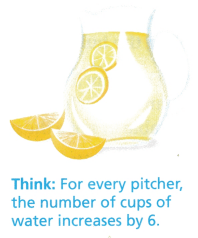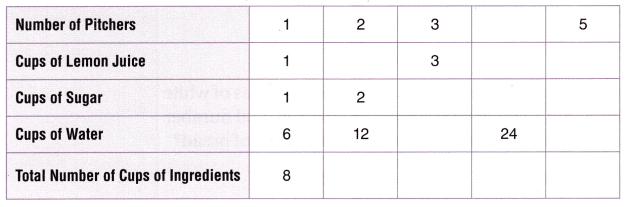So, in 5 pitchers of lemonade, there are ___ cups of lemon juice, ___ cups of sugar, and ___ cups of water. This makes a total of ___ cups of ingredients.Explanation:
Number of cups of lemon juice she uses = 1.
Number of cups of sugar she uses = 1.
Number of cups of water she uses = 6.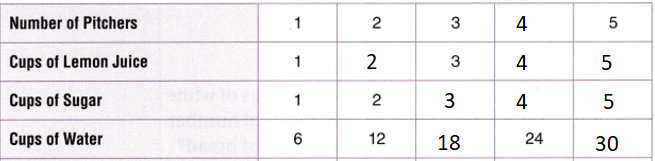Total number of cups of ingredients she will use to make 5 pitchers of lemonade = (Number of cups of lemon juice she uses + Number of cups of sugar she uses + Number of cups of water she uses) × 5
= (1 + 1 + 6) × 5
= (2 + 6) × 5
= 8 × 5
= 40.Question 2.
What pattern do you see in the total number of cups of ingredients?
It is noticed that total number of cups of ingredients is been increased doubled for each ingredients.

Explanation:Question 3.
Multi-Step Cammi needs 36 postcards. She buys 4 packages of 10 postcards. How many postcards will Cammi have left over? Explain.
Number of postcards Cammi have left over = 4.

Explanation:
Number of postcards Multi-Step Cammi needs = 36.
Number of packages she buys =  4.
Number of postcards in each package = 10.
Number of postcards Cammi have left over = (Number of packages she buys × Number of postcards in each package) – Number of postcards Multi-Step Cammi needs
= (4 × 10) – 36
= 40 – 36
= 4.

Fill in the bubble for the correct answer choice.
Question 4.
There are 5 boats in a race. Each boat has 6 rowers and 1 coach. How many people are in the race? Use the table to solve the problem.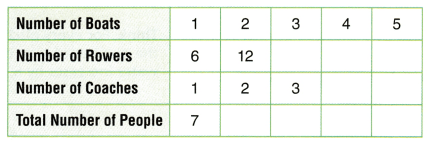(A) 7
(B) 30
(C) 12
(D) 35
Number of people are in the race = 35.
(D) 35.

Explanation:
Number of boats in a race = 5.
Number of rowers each boat has = 6.
Number of coach each boat has = 1.
Number of people are in the race = Number of boats in a race × (Number of rowers each boat has + Number of coach each boat has)
= 5 × (6 + 1)
= 5 × 7
= 35.Question 5.
Apply For each pan of bread, you need 2 cups of white flour and 1 cup of wheat flour. What is the total number of cups of flour you will need to make 4 pans of bread?
(A) 7 cups
(B) 3 cups
(C) 8cups
(D) 12 cups
Total number of cups of flour you will need to make 4 pans of bread = 12.
(D) 12 cups.

Explanation:
Number of cups of white flour needed to make each pan of bread = 2.
Number of cups of wheat flour needed to make each pan of bread = 1.
Total number of cups of flour you will need to make 4 pans of bread = 4  × (Number of cups of white flour needed to make each pan of bread + Number of cups of wheat flour needed to make each pan of bread)
= 4 × (2 + 1)
= 4 × 3
= 12.

Question 6.
Multi-Step Sheri puts 16 pictures in an album. Some pages have 2 pictures. The other pages have 4 pictures. There are 3 pages with 4 pictures. How many pages have 2 pictures?
(A) 3
(B) 2
(C) 4
(D) 1
Number of pages have 2 pictures = 4.
(C) 4.

Explanation:
Number of pictures in an album Sheri puts = 16.
Number of pictures some pages have = 2.
Number of pictures some other pages have = 4.
Number of pages with 4 pictures = 3.
Total number of pages with 4 pictures = 4 × Number of pages with 4 pictures
= 4 × 3
= 12.
Number of pages have 2 pictures = Number of pictures in an album Sheri puts – Total number of pages with 4 pictures
= 16 – 12
= 4.

Texas Test Prep
Question 7.
Each year for 8 states, the Williams family visited 6 different states on vacation. How many more states do they need to visit before they have seen all 50 states?
(A) 36
(B) 14
(C) 48
(D) 2
Number of more states do they need to visit before they have seen all 50 states = 2.
(D) 2.

Explanation:
Number of states each year for = 8.
Number of different states on vacation the Williams family visited = 6.
Number of more states do they need to visit before they have seen all 50 states = 50 – (Number of states each year for × Number of different states on vacation the Williams family visited)
= 50 – (8 × 6)
= 50 – 48
= 2.

### Texas Go Math Grade 3 Lesson 8.6 Homework and Practice Answer Key

Make a table to solve the problem.
Question 1.
Janey is making party mix. For each bowl of mix, she uses 2 cups of raisins, 1 cup of peanuts, and 4 cups of granola. What is the total number of cups of ingredients she will use to make 6 bowls of party mix?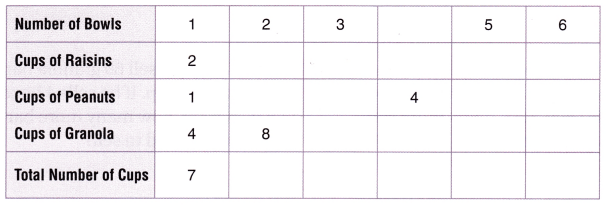So, in 6 bowls of party mix, there are __ cups of raisins, ___ cups of peanuts, and __ cups of granola.
This makes a total of ___ cups of ingredients.
Total number of cups of ingredients she will use to make 6 bowls of party mix =42.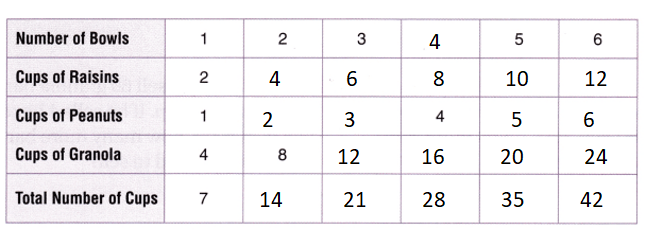Explanation:
Number of cups of raisins she uses = 2.
Number of cups of peanuts she uses = 1.
Number of cups of granola she uses = 4.
Total number of cups of ingredients she will use to make 6 bowls of party mix = 6 × (Number of cups of raisins she uses + Number of cups of peanuts she uses + Number of cups of granola she uses)
= 6 × (2 + 1 + 4)
= 6 × (3 + 4)
= 6 × 7
= 42.

Problem Solving
Question 2.
To make an apple pie, Mimi needs 2 cups of flour and 3 cups of apples. How many cups of ingredients does Mimi need to make 5 pies?
Number of cups of ingredients Mimi need to make 5 pies = 25.

Explanation:
Number of cups of flour Mimi needs = 2.
Number of cups of apples Mimi needs = 3.
Number of cups of ingredients Mimi need to make 5 pies = 5 × (Number of cups of flour Mimi needs + Number of cups of apples Mimi needs)
= 5 × (2 + 3)
= 5 × 5
= 25.

Question 3.
To make muffins, Seth needs 3 cups of mix and 1 cup of raisins. How many cups of ingredients does Seth need to make 3 batches of muffins?
Number of cups of ingredients Seth need to make 3 batches of muffins = 12.

Explanation:
Number of cups of mix Seth needs = 3.
Number of cups of raisins Seth needs = 1.
Number of cups of ingredients Seth need to make 3 batches of muffins = 3 × (3 + 1)
= 3 × 4
=  12.

Texas Test Prep
Lesson Check
Question 4.
There are 6 relay teams at a race. Each team has 4 runners and 1 captain. How many people are in the race?
(A) 11
(B) 30
(C) 24
(D) 25
Number of people are in the race = 30.
(B) 30.

Explanation:
Number of relay teams at a race = 6.
Number of runners each team has = 4.
Number of captain each team has = 1.
Number of people are in the race = Number of relay teams at a race  × (Number of runners each team has + Number of captain each team has)
= 6 × (4 + 1)
= 6 × 5
= 30.

Question 5.
Sara makes 7 fruit baskets for the senior center. She puts 3 apples and 4 pears in each basket. How many pieces of fruit are there?
(A) 14
(B) 21
(C) 49
(D) 28
Number of pieces of fruit are there = 49.
(C) 49.

Explanation:
Number of fruit baskets for the senior center Sara makes = 7.
Number of apples she puts in each basket = 3.
Number of pears she puts in each basket = 4.
Number of pieces of fruit are there = Number of fruit baskets for the senior center Sara makes × (Number of apples she puts in each basket + Number of pears she puts in each basket)
= 7 × (3 + 4)
= 7 × 7
= 49.

Question 6.
Thomas wants to collect 40 toy cars for his collection. If he has 6 boxes of 4 toy cars each, how many more cars does Thomas need?
(A) 16
(B) 24
(C) 50
(D) 34
Number of cars Thomas needs = 16.
(A) 16.

Explanation:
Number of toy cars for his collection Thomas wants to collect = 40.
Number of boxes he has = 6.
Number of toy cars in each box = 4.
Number of cars Thomas needs = Number of toy cars for his collection Thomas wants to collect – (Number of boxes he has × Number of toy cars in each box)
= 40 – (6 × 4)
= 40 – 24
= 16.

Question 7.
Vernon needs to sell 60 granola bars for his scout troop. If he sells 4 boxes of 8 bars each, how many more bars does Vernon need to sell?
(A) 48
(B) 32
(C) 28
(D) 30
Number of more bars Vernon needs to sell = 28.
(C) 28.

Explanation:
Number of granola bars for his scout troop Vernon needs to sell = 60.
Number of boxes of granola he sells = 4.
Number of bars in each box = 8.
Number of more bars Vernon needs to sell = Number of granola bars for his scout troop Vernon needs to sell – (Number of boxes of granola he sells × Number of bars in each box)
= 60 – (4 × 8)
= 60 – 32
= 28.

Question 8.
Multi-Step Jessie has 4 boxes of 8 crayons and 3 boxes of 5 markers and 2 single markers. How many more crayons than markers does Jessie have?
(A) 17
(B) 22
(C) 15
(D) 20
15 more crayons than markers Jessie have.
(C) 15.

Explanation:
Number of boxes of crayons Jessie has = 4.
Number of crayons in each box = 8.
Number of boxes of markers Jessie has = 3.
Number of markers in each box = 5.
Number of single markers = 2.
Number of crayons Jessie have = Number of boxes of crayons Jessie has × Number of crayons in each box
= 4 × 8
= 32.
Number of markers Jessie have = (Number of boxes of markers Jessie has × Number of markers in each box) + Number of single markers
=  (3 × 5) + 2
= 15 + 2
= 17.
Difference:
Number of crayons Jessie have – Number of markers Jessie have
= 32 – 17
= 15.

Question 9.
Multi-Step To make a salad, Alan needs 3 cups of lettuce and 1 cup of tomatoes. Alan makes 6 bowls of salad. How many more cups of lettuce than tomatoes does Alan need?
(A) 10 cups
(B) 12 cups
(C) 2 cups
(D) 15 cups
12 more cups of lettuce than tomatoes Alan needs.
(B) 12 cups.

Explanation:
Number of cups of lettuce Alan needs = 3.
Number of cups of tomatoes Alan needs = 1.
Number of bowls of salad Alan makes = 6.
Total number of of lettuce Alan makes = Number of cups of lettuce Alan needs × Number of bowls of salad Alan makes
= 3 × 6
= 18.
Total number of of tomatoes Alan makes = Number of cups of tomatoes Alan needs × Number of bowls of salad Alan makes
= 1 × 6
= 6.
Difference:
Total number of of lettuce Alan makes – Total number of of tomatoes Alan makes
= 18 – 6
= 12.

Scroll to Top